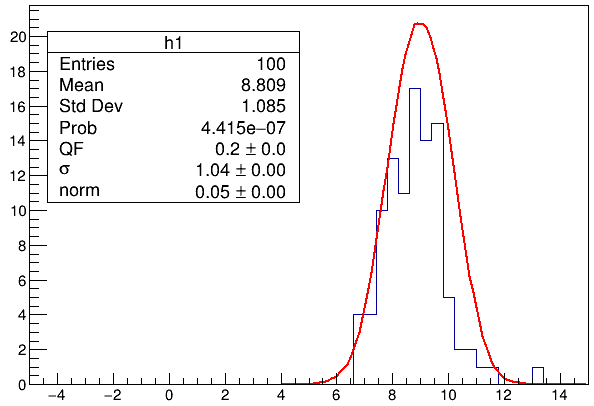# Get detector info from fitting MC to data

I need to shift and smear energies obtained from MC so that the resulting distribution matches that of data. I wrote the following code to demonstrate the idea. However, the fitting never converges. How can I solve the problem? Thanks, Jing

``````TH1F *h0;
double shift0, smear0, scale0, e;

double model(double *x, double *p)
{
double shift = p;
double smear = p;
double scale = p;

if (shift!=shift0 || smear!=smear0 || scale!=scale0) {
shift0=shift; smear0=smear; scale0=scale; // save old values
delete h0; h0 = new TH1F("h0","", 50, -5, 15); // empty histogram
// shift, smear and then scale distribution of e
for (int i=0; i<3000; i++) h0->Fill(gRandom->Gaus(e[i]*shift, smear), scale);
}
return h0->GetBinContent(int(2.5*(x+5))+1);
}

void test()
{
// create fake data histogram
TF1 fgaus("fgaus","exp(-0.5*((x-9)/1.2)**2)",4,15);
TH1F *h1 = new TH1F("h1", "", 50, -5, 15);
h1->FillRandom("fgaus", 100);

// create a set of fake MC samples
for (int i=0; i<3000; i++) e[i]=gRandom->Gaus(45,1);

// create model using fake MC samples
TF1 *f = new TF1("f", model, 4, 15, 3);
f->SetParNames("shift", "smear", "scale");
f->SetParameters(0.2,1.2,0.1);

// fit model to data
gStyle->SetOptFit(1011);
ROOT::Math::MinimizerOptions::SetDefaultMinimizer("Minuit2","Simplex");
ROOT::Math::MinimizerOptions::PrintDefault("Minuit2");

h1->Fit(f,"rlv");
h0->SetLineColor(kBlue);
h0->Draw("same");
}
``````

Yes, I see it does not converge. May be the initial parameters are too far from the final values / I guess @moneta can help.

I’ve tried different initial values, adding limits, different minuit algorithms, tolerance, etc. They help to some extent, the fitting result looks better. But I never managed to get it converge. The fitting is too sensitive to initial values and the fitting range. I am wondering if I did something fundamentally wrong.

In principle, fitting algorithms expect that the fitting function and its (first two?) derivatives with respect to parameters behave well. That’s not your case. You explicitly introduce significant “random jitter” (“random noise”) in it.

@Wile_E_Coyote, thanks for the tip. I changed `return h0->GetBinContent(int(2.5*(x+5))+1);` to `return h0->Interpolate(x);` to avoid sudden changes in the function. I also smoothed the function 5 times using `h0->Smooth(5,"R")`. The function looks pretty smooth to me by eye:But the fitting still cannot converge.

In your “`model`” function, try:

``````  // ...
double v = 0.;
for (unsigned long i = 0; i < (sizeof(e) / sizeof(double)); i++)
v += TMath::Gaus(x, e[i] * shift, smear, 1); // 0 or 1
return scale * v;
``````

@Wile_E_Coyote, it worked! Thank you!

By the way, I got

``````Info in Minuit2Minimizer::Minimize : Covar is not pos def
Minuit2Minimizer : Valid minimum - status = 5
``````

The covariance and correlation matrix elements are all zero. I don’t expect the parameters to be correlated. But do you know why all the elements are zero? And what does status 5 mean? Where can I find the explanation of status values?

Thanks, Jing

The “`status = 5`” is triggered by the “`Covar is not pos def`” (@moneta`Hesse`” and/or “`MnSeed`” failed and then all elements of the covariance and correlation matrices are zero, too?)

It is also possible that it’s because you use the `"Simplex"` minimization algorithm (which possibly does not produce the covariance matrix at all). Try, e.g.:

`ROOT::Math::MinimizerOptions::SetDefaultMinimizer("Minuit2", "Migrad"); // "Migrad" or "Combined"`

This topic was automatically closed 14 days after the last reply. New replies are no longer allowed.